# RTOS_Module 2_3_multilevelqueues.pptx

28 de May de 2023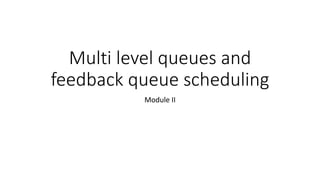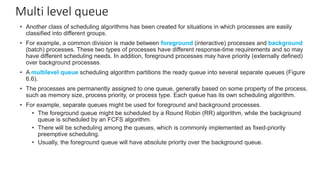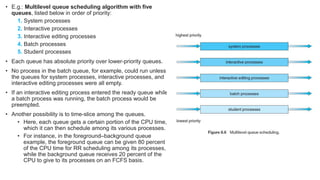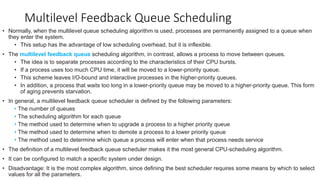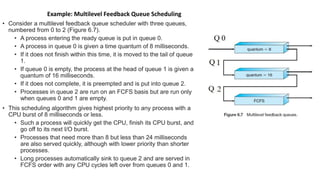1 de 5

### RTOS_Module 2_3_multilevelqueues.pptx

• 1. Multi level queues and feedback queue scheduling Module II
• 2. Multi level queue • Another class of scheduling algorithms has been created for situations in which processes are easily classified into different groups. • For example, a common division is made between foreground (interactive) processes and background (batch) processes. These two types of processes have different response-time requirements and so may have different scheduling needs. In addition, foreground processes may have priority (externally defined) over background processes. • A multilevel queue scheduling algorithm partitions the ready queue into several separate queues (Figure 6.6). • The processes are permanently assigned to one queue, generally based on some property of the process, such as memory size, process priority, or process type. Each queue has its own scheduling algorithm. • For example, separate queues might be used for foreground and background processes. • The foreground queue might be scheduled by a Round Robin (RR) algorithm, while the background queue is scheduled by an FCFS algorithm. • There will be scheduling among the queues, which is commonly implemented as fixed-priority preemptive scheduling. • Usually, the foreground queue will have absolute priority over the background queue.
• 3. • E.g.: Multilevel queue scheduling algorithm with five queues, listed below in order of priority: 1. System processes 2. Interactive processes 3. Interactive editing processes 4. Batch processes 5. Student processes • Each queue has absolute priority over lower-priority queues. • No process in the batch queue, for example, could run unless the queues for system processes, interactive processes, and interactive editing processes were all empty. • If an interactive editing process entered the ready queue while a batch process was running, the batch process would be preempted. • Another possibility is to time-slice among the queues. • Here, each queue gets a certain portion of the CPU time, which it can then schedule among its various processes. • For instance, in the foreground–background queue example, the foreground queue can be given 80 percent of the CPU time for RR scheduling among its processes, while the background queue receives 20 percent of the CPU to give to its processes on an FCFS basis.
• 4. Multilevel Feedback Queue Scheduling • Normally, when the multilevel queue scheduling algorithm is used, processes are permanently assigned to a queue when they enter the system. • This setup has the advantage of low scheduling overhead, but it is inflexible. • The multilevel feedback queue scheduling algorithm, in contrast, allows a process to move between queues. • The idea is to separate processes according to the characteristics of their CPU bursts. • If a process uses too much CPU time, it will be moved to a lower-priority queue. • This scheme leaves I/O-bound and interactive processes in the higher-priority queues. • In addition, a process that waits too long in a lower-priority queue may be moved to a higher-priority queue. This form of aging prevents starvation. • In general, a multilevel feedback queue scheduler is defined by the following parameters: • The number of queues • The scheduling algorithm for each queue • The method used to determine when to upgrade a process to a higher priority queue • The method used to determine when to demote a process to a lower priority queue • The method used to determine which queue a process will enter when that process needs service • The definition of a multilevel feedback queue scheduler makes it the most general CPU-scheduling algorithm. • It can be configured to match a specific system under design. • Disadvantage: It is the most complex algorithm, since defining the best scheduler requires some means by which to select values for all the parameters.
• 5. • Consider a multilevel feedback queue scheduler with three queues, numbered from 0 to 2 (Figure 6.7). • A process entering the ready queue is put in queue 0. • A process in queue 0 is given a time quantum of 8 milliseconds. • If it does not finish within this time, it is moved to the tail of queue 1. • If queue 0 is empty, the process at the head of queue 1 is given a quantum of 16 milliseconds. • If it does not complete, it is preempted and is put into queue 2. • Processes in queue 2 are run on an FCFS basis but are run only when queues 0 and 1 are empty. • This scheduling algorithm gives highest priority to any process with a CPU burst of 8 milliseconds or less. • Such a process will quickly get the CPU, finish its CPU burst, and go off to its next I/O burst. • Processes that need more than 8 but less than 24 milliseconds are also served quickly, although with lower priority than shorter processes. • Long processes automatically sink to queue 2 and are served in FCFS order with any CPU cycles left over from queues 0 and 1. Example: Multilevel Feedback Queue Scheduling